# Histogram¶

Title
Histogram Element
Dependencies
Matplotlib
Backends
Matplotlib
Bokeh
In :
import numpy as np
import holoviews as hv
from holoviews import opts

hv.extension('matplotlib')Histograms partition the x axis into discrete (but not necessarily regular) bins, showing counts in each as a bar. A Histogram accepts the output of np.histogram as input, which consists of a tuple of the histogram values with a shape of N and bin edges with a shape of N+1. As a simple example we will generate a histogram of a normal distribution with 20 bins.

In :
np.random.seed(1)
data = np.random.randn(10000)
frequencies, edges = np.histogram(data, 20)
print('Values: %s, Edges: %s' % (frequencies.shape, edges.shape))
hv.Histogram((edges, frequencies))

Values: 20, Edges: 21

Out: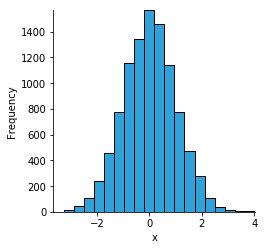The Histogram Element will also expand evenly sampled bin centers, therefore we can easily cast between a linearly sampled Curve or Scatter and a Histogram.

In :
xs = np.linspace(0, np.pi*2)
ys = np.sin(xs)
curve = hv.Curve((xs, ys))
curve + hv.Histogram(curve)

Out: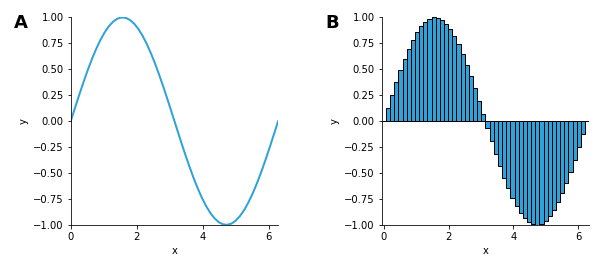Like most other elements a Histogram also supports using dim transforms to map dimensions to visual attributes. To demonstrate this we will use the bin transform to bin the 'y' values into positive and negative values and map those to a 'blue' and 'red' fill_color:

In :
hv.Histogram(curve).opts(color=hv.dim('y').bin(bins=[-1, 0, 1], labels=['red', 'blue']))

Out: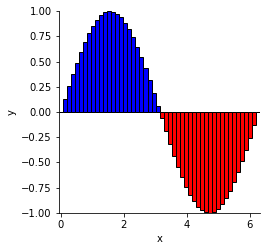The .hist method is an easy way to compute a histogram from an existing Element:

In :
points = hv.Points(np.random.randn(100,2))

points.hist(dimension=['x','y'])

Out: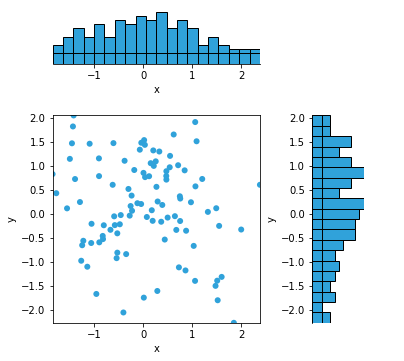The .hist method is just a convenient wrapper around the histogram operation that computes a histogram from an Element, and then adjoins the resulting histogram to the main plot. You can also do this process manually; here we create an additional set of Points, compute a Histogram for the 'x' and 'y' dimension on each, and then overlay them and adjoin to the plot.

In :
from holoviews.operation import histogram
points2 = hv.Points(np.random.randn(100,2)*2+1).redim.range(x=(-5, 5), y=(-5, 5))

xhist, yhist = (histogram(points2, bin_range=(-5, 5), dimension=dim) *
histogram(points,  bin_range=(-5, 5), dimension=dim)
for dim in 'xy')
((points2 * points) << yhist << xhist).opts(
opts.Histogram(alpha=0.3))

Out: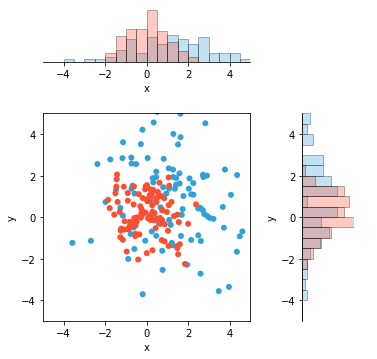For full documentation and the available style and plot options, use hv.help(hv.Histogram).